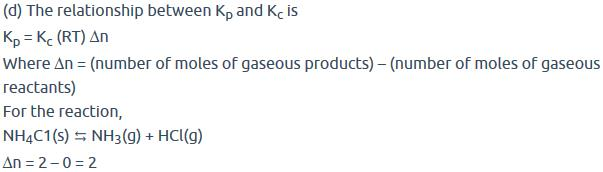Courses

# Test: Le-Chatelier's Principle (Chemical Equilibrium)

## 19 Questions MCQ Test Chemistry Class 11 | Test: Le-Chatelier's Principle (Chemical Equilibrium)

Description
This mock test of Test: Le-Chatelier's Principle (Chemical Equilibrium) for JEE helps you for every JEE entrance exam. This contains 19 Multiple Choice Questions for JEE Test: Le-Chatelier's Principle (Chemical Equilibrium) (mcq) to study with solutions a complete question bank. The solved questions answers in this Test: Le-Chatelier's Principle (Chemical Equilibrium) quiz give you a good mix of easy questions and tough questions. JEE students definitely take this Test: Le-Chatelier's Principle (Chemical Equilibrium) exercise for a better result in the exam. You can find other Test: Le-Chatelier's Principle (Chemical Equilibrium) extra questions, long questions & short questions for JEE on EduRev as well by searching above.
QUESTION: 1

### Direction (Q. Nos. 1-8) This section contains 8 multiple choice questions. Each question has four choices (a), (b), (c) and (d), out of which ONLY ONE option is correct. Q. The equilibrium which is not affected by volume change at constant temperature is

Solution:

As the number of moles of reactants and products is the same. Hence, they both will occupy equal volume at the same temperature.
Therefore, the reaction A i.e., H2 (g) +I2 (g) ⇌ 2HI (g) is not affected by change in pressure and volume.

QUESTION: 2

Solution:
QUESTION: 3

### The binding of oxygen by haemoglobin (Hb) forming (HbO2), is partially regulated by the concentration of H3O + and dissolved CO2 in blood.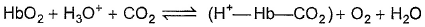Release of O2 is favoured when there is

Solution:

During exercise muscle need energy hence consume more oxygen and produce more CO2 and lactic acid gets accumulated as well. According to bohr's effect oxygen binding affinity is inversely proportional to CO2 concentration and acidity. Thus favours the dissociation​ or release of O2.

QUESTION: 4

Aerated water contains CO2 dissolved in water

CO2(g) + H2O(l)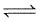H2CO3(aq)

Variation of solubility (s) with pressure (p) is shown by

Solution:

Pressure increase solubility also increase in accordance with the Henry's law and option B is the closest to this. Hence B is correct.

QUESTION: 5

Variation of log Kp with temperature. 1/T is given by for the equilibrium.
NH4HS (s)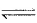NH3(g) + H2S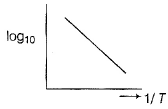Q. The equilibrium is displaced in forward side on

Solution:

The correct answer is Option A.
When ammonia is added after equilibrium is established, the partial pressure of ammonia will increase.When the temperature of an endothermic reaction is increased, the equilibrium will shift in the forward direction so that the heat is absorbed which will nullify the effect of increased temperature. Hence, the partial pressure of ammonia will increase.When the volume of the flask is increased, the pressure will decrease.

QUESTION: 6

In the following equilibrium  ABA+ + B-
AB is 10% dissociated, when [AB] = 1M

Q. What is per cent dissociation if 1 M AB is dissociated in the presence of 1 M A+

Solution:

The correct answer is option C
Let initially
AB = 1M
AB = A+  + B-
⇒1 - 0.1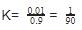K should remain same
AB ⇌ A+ + B-
1-x

QUESTION: 7

When hydrochloric acid is added to cobalt (II) nitrate solution at room temperature, the following reaction takes place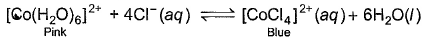Q. The solution is blue at room temperature. However, it turns pink when cooled in a freezing mixture. Based upon this information, which of the following expression is correct for the forward reaction?

Solution: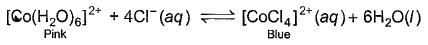According to the given information, the solution turns pink when cooled in freezing mixture. So, the equilibrium has shifted in backward direction. So we can say that the reaction is endothermic and ∆H for the reaction is greater than zero. This is because, endothermic reactions have ∆H > 0 and they proceed in reverse direction.

QUESTION: 8

Consider the following equilibrium in a closed container

N2O4 (g)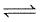2NO2(g)

At a fixed temperature, the volume of a reaction container is halved. For this change, which of the following statements holds true regarding the equilibrium constant (Kp) and degree of dissociation (α) ?

Solution:

We know that Kp depends only on Temperature. As the temp. remains constant , Kp also remains constant.
α depends on the concentration of the reactant
So as the volume of the reaction container is halved, α also changes
So option d is correct.

*Multiple options can be correct
QUESTION: 9

Direction (Q. Nos. 9-18) This section contains 10 multiple choice questions. Each question has four
choices (a), (b), (c) and (d), out of which ONE or  MORE THANT ONE  is correct.

The thermal dissociation of equilibrium of CaCO3(s) is studied under different conditions

CaCO3 (s)CaO(s) + CO2 (g)

For this equilibrium, the correct statement (s) is/are

Solution:

Heat of reaction is dependent on temperature (Kirchhoff's equation) in heterogeneous system; equilibrium constant is independent on the molar concentration of solid species. Heat of reaction is not affected by catalyst. It lowers activation energy.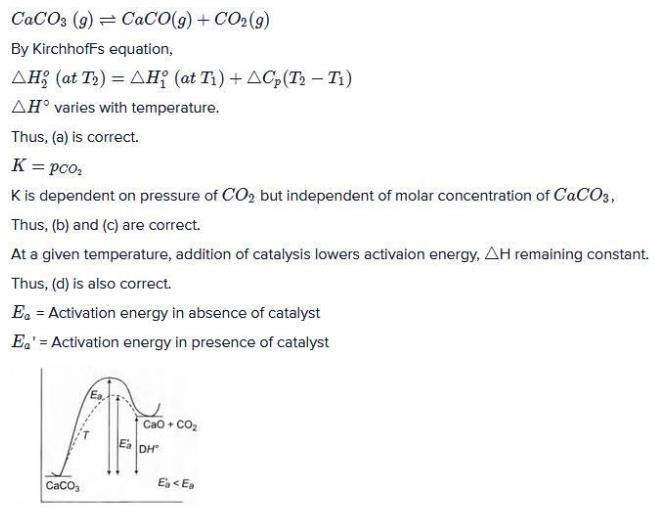*Multiple options can be correct
QUESTION: 10

For the reaction,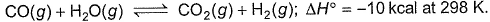Equilibrium amount of CO2(g) can be increased by

Solution:

The correct answers are option A & D
As the reaction is exothermic decreasing the temperature will move it in forward direction in order to maintain equilibrium . increasing the amount of CO would increase the reactant concentration so in order to balance it out the reaction moves forward . hence the amount of CO2 increases. so options A and D , both are correct.

*Multiple options can be correct
QUESTION: 11

Consider the following equilibrium,

N2(g) + 3H2(g)2NH3(g)

If N2(g) is added to the above mixture in equilibrium,

[IIT JEE 2006]

Solution:

Partial pressure of each component in the mixture increases on increasing concentration. Also,according to Le Chatelier's principle the reaction would shift such that kp does not change.

*Multiple options can be correct
QUESTION: 12

H2O (l)H2O(s) ; ΔH = -q

Application of pressure on this equilibrium

Solution:

The correct answers are Options B and D.

As we know that reaction is exothermic it means heat is released in the reaction so, if we apply pressure then reaction will proceed in backward direction but if there is gas phase equilibrium the reaction will shift in that direction in which less number of moles are present. If pressure increases then the ice will melt and ice gets more energy at low temp. To melt ,so it’s melting point decreases.

*Multiple options can be correct
QUESTION: 13

AgCI(s)is sparingly soluble salt,

AgCl (s)Ag+(aq) + Cl-(aq)

There is

Solution:

When ammonia is added, solubility of AgCl increases due to formation of complex salt which decreases the concentration of radicals in the product side and tus drives the reaction in forward direction.
When we add KCl common ion effect is applied in presence of common ion solubility decreases and reaction goes in backward direction.

*Multiple options can be correct
QUESTION: 14

Combustion of CO(g)can be increased in the following reaction by

2CO(g) +O2(g)2CO2(g)

Solution: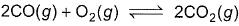For option a; with decrease in volume or with increase in pressure, reaction shifts towards less no. of moles. So, here combustion of CO will increase.
For option b; adding argon at constant volume doesn’t make any effect on equilibrium. Also, if we add argon at constant pressure, the reaction will shift towards more no. of moles and so the combustion of CO will decrease.
For option c; adding O2 will shift the reaction in forward direction (according to Le Chatelier principle), so combustion of CO will increase.
For option d; with decrease in pressure or increases in volume, reaction shifts towards more no. of moles and so combustion of CO will decrease.

*Multiple options can be correct
QUESTION: 15

increase in pressure on the following equilibrium

H2O(l)H20(g)

results is

Solution:

The correct answers are Options A and D.
As in this equilibrium, liquid converts into gas so increase in the pressure will favour reverse reaction; so formation of more H2O(l). Also, as we increase pressure boiling point of water increases.

*Multiple options can be correct
QUESTION: 16

Which of the following on the addition will cause deep red colour to disappear?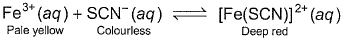Solution:

The correct answers are option A,B,C
Because of silver salt ie . silver nitrate with powerful germicidal activity
Ag+ + SCN → AgSCN↓
Hg2+ + SCN− → Hg(SCN)2
4Fe3+ + 3(COO)2−2 → 2Fe2(COO)3

*Multiple options can be correct
QUESTION: 17

Sulphuric acid is manufactured by the following reaction

2SO2(g) +O2 (g)2SO3(g) +Q

Reaction proceeds in the forward side if

Solution: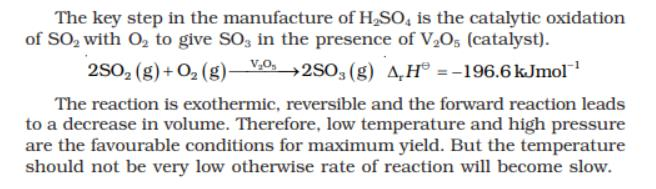*Multiple options can be correct
QUESTION: 18

For the reaction,

2SO2 (g) + O2 (g)2SO3 (g) + 188.3 KJ

the number of moles of SO3 formed is increased if

Solution:

Volume decreased

i.e p increased

no.of moles reactant more

QUESTION: 19

We know that the relationship between Kc and Kp is Kp = Kc (RT)Δn
What would be the value of Δn for the reaction NH4Cl (s) ⇔ NH3 (g) + HCl (g)

Solution: